HomeCompositio Mathematica

# Counting imaginary quadratic points via universal torsors

Published online by Cambridge University Press:  25 July 2014

Corresponding

## Abstract

A conjecture of Manin predicts the distribution of rational points on Fano varieties. We provide a framework for proofs of Manin’s conjecture for del Pezzo surfaces over imaginary quadratic fields, using universal torsors. Some of our tools are formulated over arbitrary number fields. As an application, we prove Manin’s conjecture over imaginary quadratic fields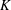$K$ for the quartic del Pezzo surface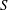$S$ of singularity type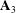${\boldsymbol{A}}_{3}$ with five lines given in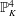${\mathbb{P}}_{K}^{4}$ by the equations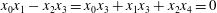${x}_{0}{x}_{1}-{x}_{2}{x}_{3}={x}_{0}{x}_{3}+{x}_{1}{x}_{3}+{x}_{2}{x}_{4}=0$ .

## MSC classification

Type
Research Article
Information
Compositio Mathematica , October 2014 , pp. 1631 - 1678

## References

Baier, S. and Browning, T. D., Inhomogeneous cubic congruences and rational points on del Pezzo surfaces, J. reine angew. Math. 680 (2013), 69151.Google Scholar
Blomer, V., Brüdern, J. and Salberger, P., On a certain senary cubic form, Proc. Lond. Math. Soc. (3) 108 (2014), 911964.CrossRefGoogle Scholar
Browning, T. D. and Derenthal, U., Manin’s conjecture for a cubic surface with D 5singularity, Int. Math. Res. Not. IMRN 2009 (2009), 26202647.Google Scholar
Browning, T. D. and Derenthal, U., Manin’s conjecture for a quartic del Pezzo surface with A 4singularity, Ann. Inst. Fourier (Grenoble) 59 (2009), 12311265.CrossRefGoogle Scholar
Baier, S. and Derenthal, U., Quadratic congruences on average and rational points on cubic surfaces, Preprint (2013), arXiv:1205.0373v2.Google Scholar
Birch, B. J., Forms in many variables, Proc. Roy. Soc. Ser. A 265 (1961/1962), 245263.CrossRefGoogle Scholar
Batyrev, V. V. and Manin, Yu. I., Sur le nombre des points rationnels de hauteur bornè des variétés algébriques, Math. Ann. 286 (1990), 2743.CrossRefGoogle Scholar
Browning, T. D., Quantitative arithmetic of projective varieties, Progress in Mathematics, vol. 277 (Birkhäuser, Basel, 2009).CrossRefGoogle Scholar
Batyrev, V. V. and Tschinkel, Yu., Rational points of bounded height on compactifications of anisotropic tori, Int. Math. Res. Not. IMRN 1995 (1995), 591635.CrossRefGoogle Scholar
Batyrev, V. V. and Tschinkel, Yu., Manin’s conjecture for toric varieties, J. Algebraic Geom. 7 (1998), 1553.Google Scholar
Batyrev, V. V. and Tschinkel, Yu., Tamagawa numbers of polarized algebraic varieties, in Nombre et répartition de points de hauteur bornée (Paris, 1996), Astérisque 251 (1998), 299340.Google Scholar
Cassels, J. W. S., An introduction to the geometry of numbers, Corrected reprint of the 1971 edition, Classics in Mathematics (Springer, Berlin, 1997).Google Scholar
Chambert-Loir, A. and Tschinkel, Yu., On the distribution of points of bounded height on equivariant compactifications of vector groups, Invent. Math. 148 (2002), 421452.CrossRefGoogle Scholar
Coste, M., An Introduction to Semialgebraic Geometry. Dottorato di ricerca in matematica/Università di Pisa, Dipartimento di Matematica. Istituti Editoriali e Poligrafici Internazionali, 2000.Google Scholar
Colliot-Thélène, J.-L. and Sansuc, J.-J., La descente sur les variétés rationnelles. II, Duke Math. J. 54 (1987), 375492.CrossRefGoogle Scholar
Davenport, H., On a principle of Lipschitz, J. Lond. Math. Soc. 26 (1951), 179183.CrossRefGoogle Scholar
de la Bretèche, R., Nombre de points de hauteur bornée sur les surfaces de del Pezzo de degré 5, Duke Math. J. 113 (2002), 421464.CrossRefGoogle Scholar
de la Bretèche, R., Répartition des points rationnels sur la cubique de Segre, Proc. Lond. Math. Soc. (3) 95 (2007), 69155.CrossRefGoogle Scholar
de la Bretèche, R. and Browning, T. D., On Manin’s conjecture for singular del Pezzo surfaces of degree 4. I, Michigan Math. J. 55 (2007), 5180.CrossRefGoogle Scholar
de la Bretèche, R. and Browning, T. D., On Manin’s conjecture for singular del Pezzo surfaces of degree four. II, Math. Proc. Cambridge Philos. Soc. 143 (2007), 579605.Google Scholar
de la Bretèche, R. and Browning, T. D., Manin’s conjecture for quartic del Pezzo surfaces with a conic fibration, Duke Math. J. 160 (2011), 169.CrossRefGoogle Scholar
de la Bretèche, R. and Browning, T. D., Binary forms as sums of two squares and Châtelet surfaces, Israel J. Math. 191 (2012), 9731012.CrossRefGoogle Scholar
de la Bretèche, R., Browning, T. D. and Derenthal, U., On Manin’s conjecture for a certain singular cubic surface, Ann. Sci. Éc. Norm. Supér. (4) 40 (2007), 150.CrossRefGoogle Scholar
de la Bretèche, R., Browning, T. D. and Peyre, E., On Manin’s conjecture for a family of Châtelet surfaces, Ann. of Math. (2) 175 (2012), 297343.CrossRefGoogle Scholar
de la Bretèche, R. and Tenenbaum, G., Sur la conjecture de Manin pour certaines surfaces de Châtelet, J. Inst. Math. Jussieu 12 (2013), 759819.CrossRefGoogle Scholar
Derenthal, U., Elsenhans, A.-S. and Jahnel, J., On the factor alpha in Peyre’s constant, Math. Comp. 83 (2014), 965977.CrossRefGoogle Scholar
Derenthal, U., On a constant arising in Manin’s conjecture for del Pezzo surfaces, Math. Res. Lett. 14 (2007), 481489.CrossRefGoogle Scholar
Derenthal, U., Manin’s conjecture for a quintic del Pezzo surface with${\boldsymbol{A}}_{2}$ singularity, Preprint (2007), arXiv:0710.1583v1.Google Scholar
Derenthal, U., Counting integral points on universal torsors, Int. Math. Res. Not. IMRN 2009 (2009), 26482699.Google Scholar
Derenthal, U., Singular del Pezzo surfaces whose universal torsors are hypersurfaces, Proc. Lond. Math. Soc. (3) 108 (2014), 638681.CrossRefGoogle Scholar
Derenthal, U. and Frei, C., Counting imaginary quadratic points via universal torsors, II, Math. Proc. Cambridge Philos. Soc. 156 (2014), 383407.CrossRefGoogle Scholar
Derenthal, U. and Frei, C., On Manin’s conjecture for a certain singular cubic surface over imaginary quadratic fields, Int. Math. Res. Not. IMRN (2014), published online, doi: 10.1093/imrn/rnu016.CrossRefGoogle Scholar
Derenthal, U. and Janda, F., Gaussian rational points on a singular cubic surface, in Torsors, étale homotopy and applications to rational points – Proceedings of the ICMS workshop in Edinburgh, 10–14 January 2011, London Mathematical Society Lecture Note Series, vol. 405 (Cambridge University Press, Cambridge, 2013), 210231.Google Scholar
Derenthal, U., Joyce, M. and Teitler, Z., The nef cone volume of generalized del Pezzo surfaces, Algebra Number Theory 2 (2008), 157182.CrossRefGoogle Scholar
Derenthal, U. and Loughran, D., Singular del Pezzo surfaces that are equivariant compactifications, Zap. Nauchn. Sem. S.-Peterburg. Otdel. Mat. Inst. Steklov. (POMI) 377 (2010), 2643; 241.Google Scholar
Derenthal, U. and Loughran, D., Equivariant compactifications of two-dimensional algebraic groups, Proc. Edinb. Math. Soc. (2), to appear, arXiv:1212.3518v2 (2013).Google Scholar
Derenthal, U. and Tschinkel, Yu., Universal torsors over del Pezzo surfaces and rational points, in Equidistribution in number theory, an introduction, NATO Sci. Ser. II Math. Phys. Chem., vol. 237 (Springer, Dordrecht, 2007), 169196.Google Scholar
Destagnol, K., La conjecture de Manin sur les surfaces de Châtelet, Master’s thesis, Université Paris 7 Diderot (2013).Google Scholar
Franke, J., Manin, Yu. I. and Tschinkel, Yu., Rational points of bounded height on Fano varieties, Invent. Math. 95 (1989), 421435.CrossRefGoogle Scholar
Frei, C., Counting rational points over number fields on a singular cubic surface, Algebra Number Theory 7 (2013), 14511479.CrossRefGoogle Scholar
Kaiser, T., Integration of semialgebraic functions and integrated Nash functions, Math. Z. 275 (2013), 349366.CrossRefGoogle Scholar
Lang, S., Algebraic number theory, Graduate Texts in Mathematics, vol. 110, second edition (Springer, New York, 1994).CrossRefGoogle Scholar
Le Boudec, P., Manin’s conjecture for a quartic del Pezzo surface with A3singularity and four lines, Monatsh. Math. 167 (2012), 481502.CrossRefGoogle Scholar
Le Boudec, P., Manin’s conjecture for two quartic del Pezzo surfaces with 3A1and A1+ A2singularity types, Acta Arith. 151 (2012), 109163.CrossRefGoogle Scholar
Le Boudec, P., Affine congruences and rational points on a certain cubic surface, Algebra Number Theory, to appear, arXiv:1207.2685v2 (2013).Google Scholar
Loughran, D., Manin’s conjecture for a singular sextic del Pezzo surface, J. Théor. Nombres Bordeaux 22 (2010), 675701.CrossRefGoogle Scholar
Loughran, D., Manin’s conjecture for a singular quartic del Pezzo surface, J. Lond. Math. Soc. (2) 86 (2012), 558584.CrossRefGoogle Scholar
Loughran, D., Rational points of bounded height and the Weil restriction, Preprint (2013),arXiv:1210.1792v2.Google Scholar
Lion, J.-M. and Rolin, J.-P., Intégration des fonctions sous-analytiques et volumes des sous-ensembles sous-analytiques, Ann. Inst. Fourier (Grenoble) 48 (1998), 755767.CrossRefGoogle Scholar
Masser, D. and Vaaler, J. D., Counting algebraic numbers with large height. II, Trans. Amer. Math. Soc. 359 (2007), 427445.CrossRefGoogle Scholar
Narkiewicz, W., Elementary and analytic theory of algebraic numbers, second edition (Springer, Berlin, 1990).Google Scholar
Peyre, E., Hauteurs et mesures de Tamagawa sur les variétés de Fano, Duke Math. J. 79 (1995), 101218.CrossRefGoogle Scholar
Peyre, E., Terme principal de la fonction zêta des hauteurs et torseurs universels, in Nombre et répartition de points de hauteur bornée (Paris, 1996), Astérisque 251 (1998), 259298.Google Scholar
Peyre, E., Points de hauteur bornée, topologie adélique et mesures de Tamagawa, in Les XXIIèmes Journées Arithmetiques (Lille, 2001), J. Théor. Nombres Bordeaux 15 (2003), 319–349.CrossRefGoogle Scholar
Peyre, E. and Tschinkel, Yu., Tamagawa numbers of diagonal cubic surfaces, numerical evidence, Math. Comp. 70 (2001), 367387.CrossRefGoogle Scholar
Salberger, P., Tamagawa measures on universal torsors and points of bounded height on Fano varieties, in Nombre et répartition de points de hauteur bornée (Paris, 1996), Astérisque 251 (1998), 91258.Google Scholar
Schanuel, S., Heights in number fields, Bull. Soc. Math. France 107 (1979), 433449.CrossRefGoogle Scholar
Schmidt, W. M., Northcott’s theorem on heights. II. The quadratic case, Acta Arith. 70 (1995), 343375.CrossRefGoogle Scholar
Skinner, C. M., Forms over number fields and weak approximation, Compositio Math. 106 (1997), 1129.CrossRefGoogle Scholar
Tanimoto, S. and Tschinkel, Yu., Height zeta functions of equivariant compactifications of semi-direct products of algebraic groups, in Zeta functions in algebra and geometry, Contemporary Mathematics, vol. 566 (American Mathematical Society, Providence, RI, 2012), 119157.CrossRefGoogle Scholar
van den Dries, L., Tame topology and o-minimal structures, London Mathematical Society Lecture Note Series, vol. 248 (Cambridge University Press, Cambridge, 1998).CrossRefGoogle Scholar
Widmer, M., Lipschitz class, narrow class, and counting lattice points, Proc. Amer. Math. Soc. 140 (2012), 677689.CrossRefGoogle Scholar

### Full text views

Full text views reflects PDF downloads, PDFs sent to Google Drive, Dropbox and Kindle and HTML full text views.

Total number of HTML views: 1
Total number of PDF views: 59 *
View data table for this chart

* Views captured on Cambridge Core between September 2016 - 3rd December 2020. This data will be updated every 24 hours.

Access

Hostname: page-component-79f79cbf67-t2s8l Total loading time: 3.606 Render date: 2020-12-03T05:34:26.916Z Query parameters: { "hasAccess": "1", "openAccess": "0", "isLogged": "0", "lang": "en" } Feature Flags last update: Thu Dec 03 2020 05:05:55 GMT+0000 (Coordinated Universal Time) Feature Flags: { "metrics": true, "metricsAbstractViews": false, "peerReview": true, "crossMark": true, "comments": true, "relatedCommentaries": true, "subject": true, "clr": false, "languageSwitch": true }

# Send article to Kindle

Note you can select to send to either the @free.kindle.com or @kindle.com variations. ‘@free.kindle.com’ emails are free but can only be sent to your device when it is connected to wi-fi. ‘@kindle.com’ emails can be delivered even when you are not connected to wi-fi, but note that service fees apply.

Find out more about the Kindle Personal Document Service.

Counting imaginary quadratic points via universal torsors
Available formats
×

# Send article to Dropbox

To send this article to your Dropbox account, please select one or more formats and confirm that you agree to abide by our usage policies. If this is the first time you use this feature, you will be asked to authorise Cambridge Core to connect with your <service> account. Find out more about sending content to Dropbox.

Counting imaginary quadratic points via universal torsors
Available formats
×

# Send article to Google Drive

To send this article to your Google Drive account, please select one or more formats and confirm that you agree to abide by our usage policies. If this is the first time you use this feature, you will be asked to authorise Cambridge Core to connect with your <service> account. Find out more about sending content to Google Drive.

Counting imaginary quadratic points via universal torsors
Available formats
×
×